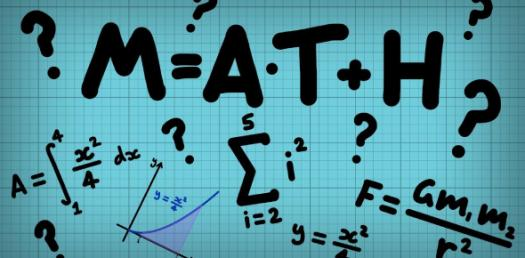# Can You Find And Match The Value?

4 Questions | Total Attempts: 181SettingsAt times you are asked to find the term number when a value is first reached or exceeded. This involves using page 1. 3 to find the value, and then match the value to the term number. Note: If a term must 'exceed' 200, then it must be ABOVE 200.

Related Topics
• 1.
Above is a rule for an arithmetic series. Find the term number where the sum of this sequence first exceeds 0.
• 2.
Above are the first term and common difference for an arithmetic sequence. Find the term number where the value first exceeds 0.
• 3.
Above is a rule for a sequence. Find the term number of the sequence which first exceeds 45.
• 4.
Above is a rule for a sequence. Find the term number of the sequence which first exceeds 110.
• 5.
Above is a rule for a sequence. Find the term number of the sequence which first exceeds 140.
• 6.
Above is a rule for a sequence. Find the term number of the sequence which first exceeds 80.
• 7.
Above is a rule for a sequence. Find the term number of the sequence which first exceeds 20.
• 8.
Above are the first four terms for an arithmetic sequence. Find the term number where the value for the SERIES first exceeds 100.
• 9.
Above is a rule for a sequence. Find the term number of the sequence which first exceeds 100.
• 10.
Above is a rule for an arithmetic series. Find the term number where the sum of this sequence first exceeds 1000.
• 11.
Above is a rule for an arithmetic series. Find the term number where the sum of this sequence first exceeds 300.
• 12.
Above is a rule for an arithmetic series. Find the term number where the sum of this sequence first exceeds 255.
• 13.
Above is a rule for an arithmetic series. Find the term number where the sum of this sequence first exceeds 200.
• 14.
Above is a rule for an arithmetic series. Find the term number where the sum of this sequence first exceeds 100.
• 15.
Above is a rule for an arithmetic series. Find the term number where the sum of this sequence first exceeds 70.
• 16.
Above are the first term and common difference for an arithmetic sequence. Find the term number where the value first exceeds 100.
• 17.
Above are the first term and common difference for an arithmetic sequence. Find the term number where the value first exceeds 50.
• 18.
Above are the first term and common difference for an arithmetic sequence. Find the term number where the value first exceeds 20.
• 19.
Above are the first term and common difference for an arithmetic sequence. Find the term number where the value first exceeds 30.
• 20.
Above are the first term and common difference for an arithmetic sequence. Find the term number where the value first exceeds 10.
• 21.
Above are the first four terms for an arithmetic sequence. Find the term number where the value for the SERIES first exceeds 50.
• 22.
Above are the first four terms for an arithmetic sequence. Find the term number where the value for the SERIES first exceeds 150.
• 23.
Above are the first four terms for an arithmetic sequence. Find the term number where the value for the SERIES first exceeds 1000.
• 24.
Above are the first four terms for an arithmetic sequence. Find the term number where the value for the SERIES first exceeds 500.
• 25.
Above are the first four terms for an arithmetic sequence. Find the term number where the value for the SERIES first exceeds 400.
• 26.
Above are the first four terms for an arithmetic sequence. Find the term number where the value for the SERIES first exceeds 200.